SLUSD37E October   2017  – November 2019

PRODUCTION DATA.

1. Features
2. Applications
3. Description
1.      Device Images
4. Revision History
5. Device Comparison Tables
6. Pin Configuration and Functions
7. Specifications
8. Detailed Description
1. 8.1 Overview
2. 8.2 Functional Block Diagram
3. 8.3 Feature Description
1. 8.3.1 CrM/DCM Control Principle
2. 8.3.2 Line Voltage Feed-Forward
3. 8.3.3 Valley Switching and CrM/DCM Hysteresis
4. 8.3.4 Transconductance Amplifier with Transient Speed-up Function
5. 8.3.5 Faults and Protections
6. 8.3.6 High-Current Driver
4. 8.4 Controller Functional Modes
9. Application and Implementation
1. 9.1 Application Information
2. 9.2 Typical Application
1. 9.2.1 Design Requirements
2. 9.2.2 Detailed Design Procedure
1. 9.2.2.1 Custom Design With WEBENCH® Tools
2. 9.2.2.2 Power Stage Design
3. 9.2.2.3 ZCD/CS Pin
4. 9.2.2.4 VOSNS Pin
5. 9.2.2.5 Voltage Loop Compensation
3. 9.2.3 Application Curves
10. 10Power Supply Recommendations
11. 11Layout
1. 11.1 Layout Guidelines
2. 11.2 Layout Example
12. 12Device and Documentation Support
13. 13Mechanical, Packaging, and Orderable Information

• DBV|6

#### 9.2.2.2.1 Boost Inductor Design

The minimum equivalent resistance presented, to the Line, by the input of the Boost PFC stage changes according to the current Line Feed-Forward setting. RInEqMin0 and RInEqMin1 present the minimum equivalent input resistance for the first two Line Feed-Forward levels.

Equation 13.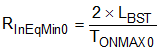Equation 14.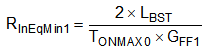Equation 15 calculates the maximum input power that can be drawn from a given Line voltage. The maximum input power is set to 110% of POutMax to account for power stage efficiency.

Equation 15.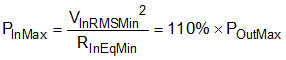Equation 16 calculates the Boost inductance value required to ensure that maximum load can be delivered from minimum Line voltage.

Equation 16.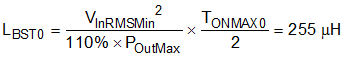Ensure that POutMax can be delivered from the lowest Line voltage for GFF1. Use Equation 17 to calculate the required Boost inductor value .

Equation 17.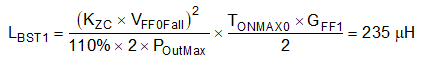Choose the lower of the two values calculated in Equation 16 and Equation 17 (LBST0 and LBST1). Using a smaller inductance value compromises light load efficiency. A larger inductance value cannot deliver the required maximum load power (POutMax) across the required range of Line voltage.

Choose a Boost inductor value of 200 µH, considering a tolerance of 10%. In order to deliver maximum load power the inductor must be able to operate with a peak current that is greater than both ILPk0 and ILPk1

Equation 18.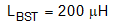Equation 19.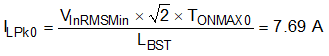Equation 20.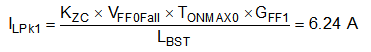Equation 21.Use Equation 22 to calculate a current sense resistance that ensures the required peak inductor current (ILPk) does not cause early termination of the TON period.

Equation 22.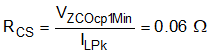Achieve this amount of resistance by connecting three resistors in parallel.

Equation 23.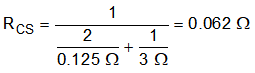Use Equation 24 to calculate an inductance value that allows a saturation current above the maximum Ocp1 current limit value.

Equation 24.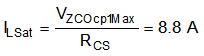Maximum current in the power components flows while delivering maximum load when supplied from minimum Line voltage. In this condition, the UCC28056 controller always operates in transition mode (CrM). shows the inductor current waveforms for ideal CrM operation.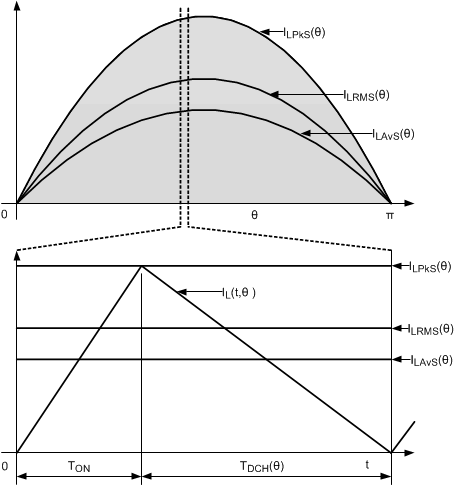Figure 27. Ideal Transition Mode (CrM) Inductor Current

Equation 25 describes the he Boost inductor RMS current over a single switching cycle, at angle θ through the Line half-cycle.

Equation 25.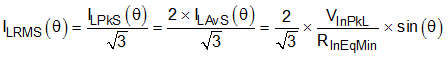Equation 26 describes the Boost inductor RMS current over a complete Line cycle.

Equation 26.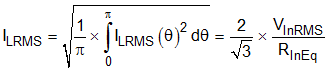Maximum Boost inductor RMS current occurs at minimum Line voltage and maximum input power.

Equation 27.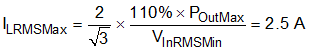Based upon the inductor requirements, a custom magnetic can be designed, or a suitable catalogue controller selected.

### Table 2. Inductor Requirements

Description Value Unit
Inductance 200 µH
RMS Current 2.5 A
Saturation Current 8.8 A